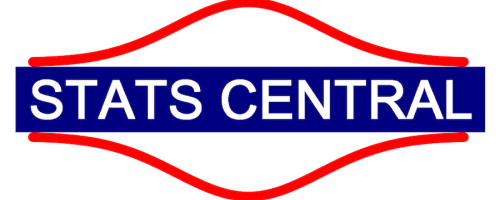# Introduction to Regression Modelling in R

Mon, 26/08/2019 - 09:00 to Wed, 28/08/2019 - 17:00The core outcome from this course is to recognise that most statistical methods you use can be understood under a single framework, as special cases of (generalised) linear models. Learning statistical methods in a systematic way, instead of as a "cookbook" of different methods, enables you to take a systematic approach to key steps in analysis (like assumption checking) and to extend your skills to handle more complex situations you might encounter in the future (random factors, multivariate analysis, choosing between a set of competing models).

This three-day short course is aimed at applied researchers with prior experience using R and familiar with introductory statistics tools - you should know about the t-test, linear regression, analysis of variance and know something about orthogonal and nested designs.  If you have not used R before, we strongly recommend you learn basic features of R and how to use the RStudio interface to R before this course.  If you need to revise introductory statistics material, you should attend the Introductory Statistics for Researchers course from 21st to 22nd August, 2019 and then take this regression course later, as the material in the Introductory Statistics for Researchers course is taken as assumed knowledge for this regression course.

### Course Outline

An outline of topics included in this regression course is below.

Linear regression
Simple linear regression, including assumptions, influential observations and inference
Equivalence of two-sample t-test and linear regression

Linear models with multiple predictor variables
Multiple regression
Analysis of variance (ANOVA), including multiple comparisons

More linear models
Paired and blocked designs
ANCOVA
Factorial experiments
Interactions in regression

Model selection
Cross-validation
Information criteria (AIC, BIC)
Penalised estimation (LASSO)

Mixed effects models
Random effects
Linear mixed effects models
Inference for mixed models, including likelihood ratio tests, parametric bootstrap, hypothesis tests, confidence intervals
Correlated random effects

Wiggly models
Spline smoothers, including diagnostics and interactions

Generalised linear models (GLMs)
Examples of GLMs
Fitting GLMs and checking assumptions, including mean-variance relationship
Inference
Extensions - offsets, zero-inflated models

You will need to bring your own laptop! We will sort out internet access for you.

Course fees include morning tea and lunch.

You will receive a certificate of completion for the course.

Registration closing date

#### Event details

50
Seat availability
UNSW Student/Staff \$300/\$600, External student \$1,125, External non-student \$1,500

#### Location

UNSW Business School (E12), Room 119
UNSW Kensington Campus

Open map

#### Key contact

stats.central@unsw.edu.au